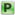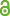Hauptmenü
• Autor
• Horvat, Alen
• Pourovskii, Leonid
• Aichhorn, Markus
• Mravlje, Jernej
• TitelTheoretical prediction of antiferromagnetism in layered perovskite Sr2TcO4
• Datei
• Persistent Identifier
• Erschienen inPhysical Review / B
• Band95
• Erscheinungsjahr2017
• Heft20
• Seiten205115
• LicenceCC-BY
• Zugriffsrechte• AbstractWe theoretically investigate the magnetic properties of $$Sr_2TcO_4$$ , a 4d transition-metal layered perovskite of the $$K_2NiF_4$$ type with half-filled $$t_{2g}$$ states. The effect of local Coulomb repulsion between the $$t_{2g}$$ orbitals is included within the density-functional theory (DFT) + U and DFT + dynamical mean-field theory (DMFT) methods. The DFT + DMFT predicts paramagnetic $$Sr_2TcO_4$$ to be close to the Mott insulator-to-metal transition, similarly to the cubic compound $$SrTcO_3$$ . The intersite exchange interactions computed within the DFT + DMFT framework point to a strong antiferromagnetic coupling between the neighboring Tc sites within the layer. We then evaluate the Néel temperature $$T_N$$ within a classical Monte Carlo approach including dipolar interactions, which stabilize the magnetic order in the frustrated $$K_2NiF_4$$ lattice structure. Our approach is validated by applying it to a set of layered and cubic perovskites, for which we obtain $$T_N$$ in fair agreement with experiment. Within the same approach we obtain the $$T_N$$ of $$Sr_2TcO_4$$ to be about 450 K. We explore also the effect of anisotropy in exchange interactions due to spin-orbit coupling. These lead to a somewhat higher transition temperature, 550 K.